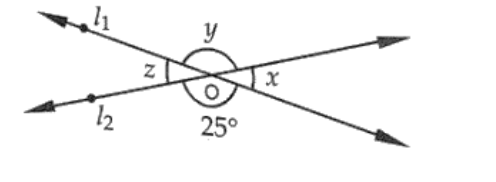# In the given fig, find the values of x, y and z.Question:

In the given fig, find the values of x, y and z.Solution:

From the given figure

y = 25     [Vertically opposite angles are equal]

Now ∠x + ∠y = 180°

[Linear pair of angles]

x = 180 - 25

x = 155

Also,

z = x = 155    [Vertically opposite angles]

y = 25

z = 155